﻿

### Translation planes admitting a linear Abelian group of order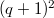$(q+1)^2$.

#### Abstract

Translation planes of order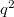$q^2$ and spread in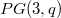$PG(3,q)$, where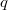$q$ is an odd prime power and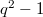$q^2-1$ has a$p$-primitive divisor, that admit a linear Abelian group of order$(q+1)^2$ containing at most three kernel homologies are shown to be associated to flocks of quadratic cones.

DOI Code: 10.1285/i15900932v29n1supplp59

Keywords:
Translation plane; flock of quadratic cone; homologies

Full Text: PDF